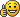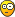Page 1 of 1Posted: Sun Mar 20, 2016 8:40 pm
Can somone give me a step by step or a brief explanation how to make a gravity pad? (point A: go up Point B: Regular gravity)Posted: Mon Mar 21, 2016 12:47 am
Box with thruster?Posted: Wed May 11, 2016 8:03 pm
Very simple:

e.other.postStep = (e)=>{
vel = vel + [0,9.81*2]/sim.frequency
}
it adds the default gravity force times 2 to the object, 1 time to negate the default gravity, and another time to apply inverted gravity. It also works with sideways gravity, check some of my scenes for examplesPosted: Sun May 15, 2016 11:15 am
it's better use gravityStrength in formula instead of value.Posted: Wed Nov 02, 2016 4:47 am
Why divide by sim.frequency?Posted: Wed Nov 02, 2016 10:15 am
Xray wrote:Why divide by sim.frequency?Because you add velocity every step. More frequent you do - less delta velocity you need.
Acceleration is m/s^2 so to get V (m/s) you need to multiply to time (s). Frequency is (s^-1) so you need to divide.

btw that code is better:

Code: Select all
`(e)=>{    vel = vel + [ - math.sin(sim.gravityAngleOffset), math.cos(sim.gravityAngleOffset)] * Sim.gravityStrength / Sim.frequency}`Posted: Wed Nov 02, 2016 5:54 pm
Kilinich wrote:Because you add velocity every step. More frequent you do - less delta velocity you need.
Acceleration is m/s^2 so to get V (m/s) you need to multiply to time (s). Frequency is (s^-1) so you need to divide.

That makes sense. Thanks!Posted: Sat Dec 10, 2016 12:05 am
@Xray

Formula for accelleration:
v=a*t
so delta-v = a*delta-t
and delta-t gets smaller the more frequent the frames get, so divide by sim.frequency to get the proper value per second. After all, if the frequency is 60, and you divide t by 60, you will add 60 times 1 60th of t to the value, and after a full second, it's 1*t in total. Makes sense rite?Posted: Sat Dec 10, 2016 6:05 am
Yes sir!Posted: Sun Mar 10, 2019 1:06 pm

e.other.density = 0.005

e.other.density = 2 (Change the number if the original density was different)Posted: Mon Mar 11, 2019 8:21 am
Kilinich wrote:
Xray wrote:Why divide by sim.frequency?Because you add velocity every step. More frequent you do - less delta velocity you need.
Acceleration is m/s^2 so to get V (m/s) you need to multiply to time (s). Frequency is (s^-1) so you need to divide.

btw that code is better:

Code: Select all
`(e)=>{    vel = vel + [ - math.sin(sim.gravityAngleOffset), math.cos(sim.gravityAngleOffset)] * Sim.gravityStrength / Sim.frequency}`

I was playing around with kilinich's code (shown above) and it caused some very odd movements of a circle and a box. Please take a look at the simple scene that I created and tell me what you think is causing the strange behavior that I have experienced.Posted: Mon Mar 11, 2019 10:15 pm
What you are experiencing there is a common error of algodoo. Attempting to apply velocity to a fixed object is nonsensical, so what algodoo seems to do is give the entire static scenery(everything with body=0) that velocity when doing collision calculation, thus causing everything to bounce up in the sky when touching anything fixed to the ground. This is only a theory of mine, but seems to make sense(considering this behavior is quite nonsensical and quite a dumb oversight, but oh well, thyme is "experimental")Posted: Tue Mar 12, 2019 12:00 am
FRA32 wrote:Attempting to apply velocity to a fixed object is nonsensical,

Really? I thought users did that often in scenes, such as when a rocket lifts off the launch pad. Its velocity is zero while at rest, then suddenly gets a huge boost in the +Y direction during liftoff.

FRA32 wrote: what algodoo seems to do is give the entire static scenery(everything with body=0) that velocity when doing collision calculation

The bouncing objects in my scene do not have body = 0. It is my understanding that all objects that have body = 0 are objects that are glued to the background. All other objects not glued to the background have body > 0.
I have been using Algodoo and Thyme scripting since 2012, and I have never witnessed this behavior until now. Since kilinich's code is responsible for this, I hope that he will chime in and give his opinion about what might be causing this strange behavior.

Thanks!Posted: Thu Mar 14, 2019 7:13 am
Fra32 - I misunderstood what you meant when you said "Attempting to apply velocity to a fixed object is nonsensical". I did not realize that what you meant was a GLUED object. You are correct that it does not make sense to apply velocity to an object that is glued to the background. When you do that, it makes other objects move with a velocity even though their velocity was not changed by the user or by the Thyme script. Most assuredly a strange bug!

Sorry for misunderstanding your comment, and thinks for your input which is always appreciated.Posted: Thu Mar 14, 2019 8:35 pm
You could imagine it like this:

If you had a glued object with the code vel = [1,0], then it would try to move with that velocity, but fails to do so since it belongs to bodygroup 0(glued to background). What algodoo does now is take ALL The objects that have this body group(fixated objects, glued objects, planes) and pretends that all of these are having said velocity of [1,0] without actually moving them. The consequence is the following: Whenever a moving object touched, say, the ground plane, or any glued object for that matter, algodoo calculates "oh, this moving object fell into an object that is moving 1 to the right per second, so friction/collision should transfer some of that velocity over" and what happens? The object bounces to the right, despite hitting a staitonary object. As the velocity of the glued parts does not change from collisions, this state is permanent until you forcefully set the velocity of any glued object to [0,0] again, or add a new glued object to the scene, thus resetting the "fake velocity" to 0 again.Posted: Thu Mar 14, 2019 11:24 pm
That makes sense. Thanks again, FRA32!Posted: Fri Mar 15, 2019 12:22 am
Yeah, gravity pads are a tricky one! Many people I know on Algodoo use this script:
Code: Select all
`(e)=>{    e.other.density = 0.005}`

However, I don't find the gravity for those to be realistic. (I call them float pads for that reason!) I use a more advanced version of it which has the added bonus of setting the gravity to what angle you want!
Code: Select all
`(e)=>{    e.other.poststep = (e)=>{        vel = vel + [ - math.sin(3.1415925), math.cos(sim.gravityAngleOffset)] * (Sim.gravityStrength) * 2 / Sim.frequency    }}`

Can't believe Algoryx decided to end development back in 2013...Posted: Fri Mar 15, 2019 12:56 am